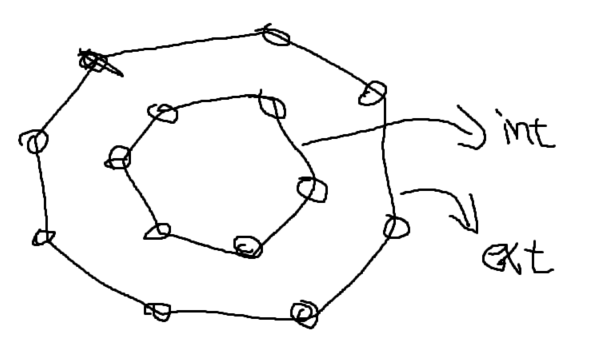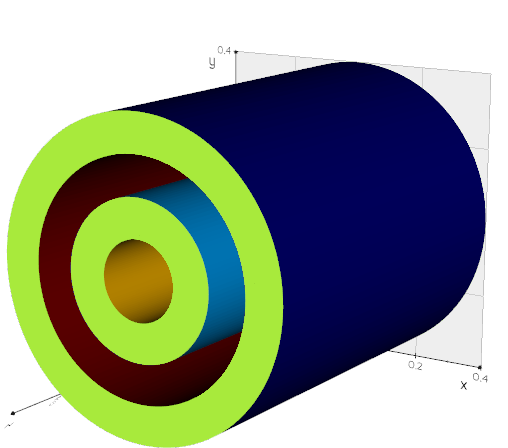# How to distinguish exterior and interior contour from vtkCutter?

Say I have a tube. I can use `vtkCutter` to get the intersection between the tube and a plane as vtkPolyData. This polydata contains two disjoint polygon.I can use `vtkConnectivityFilter` to distinguish the points/cells from disjoint polygons. But what is a robust way to tell which one represents the exterior/interior contour?

Thanks,
Cam

I would use the cell normals wrt axis to distinguish them, eg.:

``````from vedo import *
d1 = Disc(r1=0.1, r2=0.2)
d2 = Disc(r1=0.3, r2=0.4)
tube = merge(d1,d2).extrude(1).flat().computeNormals()
centers = tube.cellCenters()[:,(0,1)]
normals = tube.celldata["Normals"][:,(0,1)]
value = np.sum(centers*normals, axis=1)
tube.cmap('jet', value, on='cells').show(axes=1)
``````what does this line do? Can you elobrate? Thanks.

the scalar product of the vector position to the normal (actually a square is missing but it’s just to give the idea).